2018/07/30 16:03

# 一. 算法基础

### 大 O

（差不多是到达终点最多可能使用的运行次数）

### 归并排序的复杂度

（分治思想）复杂度 T(n) = 2T(n/2) +O(n) = O(nlogn)

void Merge(int a[],int left ,int mid,int right){
int i=left,j=mid+1,n=0,length=right-left;
//i开始为左半部分最左边，j为右半部分最左边。temp数组是从下标0开始存数。
while(i<=mid&&j<=right){
if(a[i]>a[j]){ //左边比右边大。
temp[n++]=a[j++];
num+=mid-i+1; //从i到mid都是比a[j]大。
}
else temp[n++]=a[i++];
}
if(i>mid){
//因为前面的判断条件是i<=mid，这里说明的是左边全部填满了，那就是填右边了。
while(j<=right) temp[n++]=a[j++];
}
else{
while(i<=mid) temp[n++]=a[i++];
}
for(int k=0;k<=length;k++){ //最后赋值到原数组必须要有的。
a[left+k]=temp[k];
}
}

void mergesort(int a[],int left,int right){
if(left<right){
int mid=(left+right)/2;
mergesort(a,left,mid);
mergesort(a,mid+1,right);
Merge(a,left,mid,right);
}
}

### 什么是 STL？

STL（Standard Template Library）是 C++ 标准模板库，里面提供了大量模板。

### 队列 （先进先出）

queue中元素在内存中不一定连续。

q.push(x) 向队列 q 末尾加入元素 x 。

q.front() 返回队列 q 开头元素。q.back() 返回队列 q 末尾元素。

q.size() 返回队列 q 元素个数。q.empty() 返回队列 q 是否为空。

### 栈 （后进先出）

stack中元素在内存中不一定连续。

t.top() 返回 t 栈顶元素。t.pop() 弹出 t 栈顶元素。

t.push(x) 将元素 x 压入 t 栈顶。

### vector （不定长数组）

vector中元素在内存中连续，支持随机寻址（任意询问任一元素）。

v.push_back(x) 将元素 x 压入 v 末尾。v.clear() 清空 v 中所有元素。

v.begin() 指向 vector 中0号位置的元素的指针地址。

v.end() 指向 vector 中最后一个元素的下一个的指针地址。

Q：迭代器 it ？（int类型）

A：指向内存中的地址。定义方式 vector<int> ：：iterator it；

it++；//地址向后移一位 （ it+=2 在目前的部分版本中是可以的 ）

### priority_queue （优先队列）

（ 注意有三个元素要写，vector<int>无意义，但要写；或者只写前面的一个也可以 ）

struct node{ //默认大根堆
int x,y; //先按和排序，再按x排序
bool operator<(const node &v) const {
if(x+y!=v.x+v.y) return x+y < v.x+v.y;
return x<v.x;
} //重载之后变为从小到大排序
};

priority_queue<node> q;

q.top() 返回 q 中堆顶。q.pop() 弹出 q 中堆顶。

q.push(x) 将 x 压入 q 中。

### set （去重并已经排序的集合）

set支持插入、删除、查找元素，并且支持查询大于（等于）某值的最小元素。

s.insert(x) 将 x 加入集合 s 中。s.begin() 返回集合 s 第一个元素的迭代器。

s.end() 返回集合最后一个元素下一个位置的迭代器。

//↑↑↑已去重集合
for(set<int> ::iterator it=s.begin(); it!=s.end(); it++)
cout<<(*it)<<endl;

//↓↓↓未去重集合
for(multiset<int> ::iterator it=s.begin(); it!=s.end(); it++)
cout<<(*it)<<endl;

### multiset （可重集合）

multiset与 set 相同，但允许集合中有多个相同元素。

### map （散列表&&映射关系）

map是一种关联容器，提供一对一映射处理的能力。

map<int,int> a;

int main(){
a=5; a=6;
//pair<int,int> (2,5)
cout << (*a.find(2)).first << endl;
cout << (*a.find(2)).second << endl;
cout << (a.find(2)==a.end()) << endl;
//a.find(2)返回迭代器所表现的位置
}

### pair （将2个数据组合成一个数据）

// 排序时，默认先比较第一关键字，再比较第二关键字。

typedef pair<int,int> mp;
mp b={mp(2,4),mp(3,5),mp(1,5),mp(2,3)};

int main(){
sort(b,b+4);
for(int i=0;i<4;i++)
cout<<b[i].first<<" "<<b[i].second<<endl;
}

### stl中复杂度的比较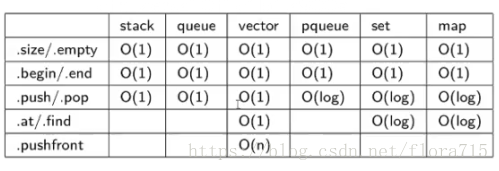### auto （自动寻找元素类型）

auto it = v.begin(); //用auto赋初始值，自动寻找it类型
for(auto x:v) cout<<x<<endl; //按顺序从前到后输出

### 二分 （logn）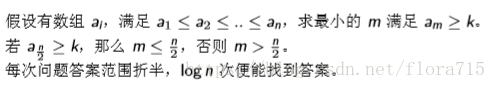vector / set / multiset 都提供了 lower_bound 函数。

lower_bound(b, e, x) 会返回 [b,e) 中第一个值不小于 x 的地址

upper_bound(b, e, x) 会返回 [b,e) 中第一个值大于 x 的地址。（左闭右开）

#include <algorithm>//必须包含的头文件
#include <stdio.h>
using namespace std;

int main(){
int n,a,m;
int left,right,i;
scanf("%d",&n);//设初始数组内元素有n个
for(i=0;i<n;i++) scanf("%d",&a[i]);
scanf("%d",&m);//插入的数为m

left = upper_bound(a,a+n,m)-a;//按从小到大，m最多能插入数组a的哪个位置
right = lower_bound(a,a+n,m)-a;//按从小到大，m最少能插入数组a的哪个位置

printf("m最多能插入数组a的%d\n",left);
for(i=0;i<left;i++) printf("%d ",a[i]);
printf("%d ",m);
for(i=left;i<n;i++) printf("%d ",a[i]);

printf("\n");

printf("m最少能插入数组a的%d\n",right);
for(i=0;i<right;i++) printf("%d ",a[i]);
printf("%d ",m);
for(i=right;i<n;i++) printf("%d ",a[i]);
return 0;
}

### 二维前缀和

【分析】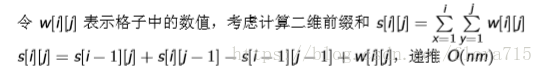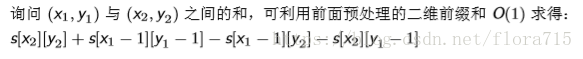【差分】若已知前缀和 s，求原数组 w 的过程叫做差分

### 高位前缀和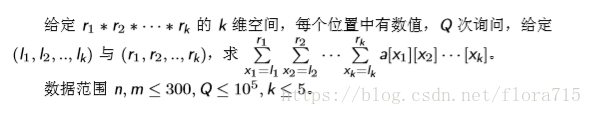【分析】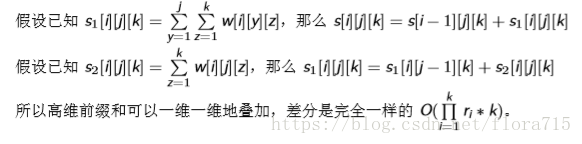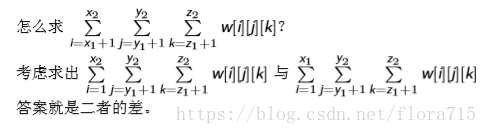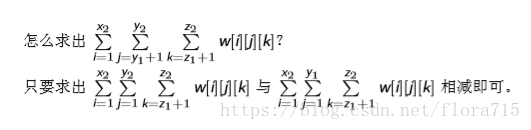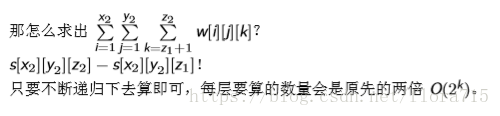# 二. 例题

### 1. tyvj 1359 收入计划 （二分答案）

【分析】考虑检验能否将数组分成 m 段使得每一段的和都不超过 x。#include <stdio.h>
#include <algorithm>
#include <string.h>
#include <iostream>
using namespace std;

const int N=100005;
int n,m,data[N],l,r,mid,ans,Max,sum;

bool check() {
int cnt=1,sum=0;
for (int i=1;i<=n;i++){
sum+=data[i]; //sum是滚动的
//尽量加在前一组，加不了，再重开一组
if (sum>mid) cnt++,sum=data[i];
if (cnt>m) return false;
}
return true;
}

int main() {
cin>>n>>m;
for (int i=1;i<=n;i++) {
cin>>data[i];
Max=max(Max,data[i]);
sum+=data[i];
}
l=Max; r=sum;
while (l<=r) {
mid=(l+r)>>1;
if (check()) ans=mid,r=mid-1;
else l=mid+1;
}
cout<<ans<<endl;
return 0;
}
View Code

### 2. 51nod 1105 第 K 大的数

【分析】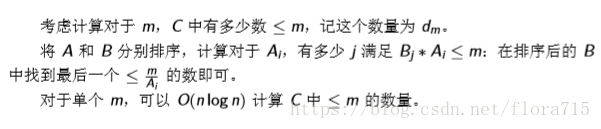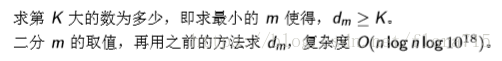n*log(n)的算法，二分里面再套一个二分。

（代码中是求的c中有多少数>=x）#include <iostream>
#include <cstdio>
#include <cstring>
#include <cstdlib>
#include <algorithm>

using namespace std;
typedef long long LL;
const int MAXN = 5e4+5;
LL a[MAXN], b[MAXN];

LL Judge(LL x, int n){ //找 a[i]*b[j]>=x 的数目
LL sum = 0, tp;
for(int i=n-1; i>=0; i--){ //枚举a数组，二分b数组
if(x % a[i]) tp = x/a[i]+1;
else tp = x/a[i];
int tmp = lower_bound(b,b+n,tp)-b;
sum += n-tmp;
if(sum == 0) break;
}
return sum;
}

int main(){
int n, k;
while(~scanf("%d%d",&n,&k)){
for(int i=0; i<n; i++)
scanf("%I64d%I64d",&a[i],&b[i]);
sort(a, a+n); sort(b, b+n);
LL l = a*b, r = a[n-1]*b[n-1];
while(l <= r){ //二分答案
LL mid = (l+r)>>1;
LL tmp = Judge(mid, n);
if(tmp < k) r = mid-1;
else l = mid+1;
}
printf("%I64d\n",l-1);
}
return 0;
}
View Code

### 3.分数规划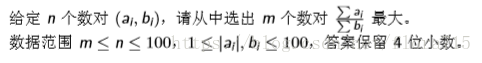【分析】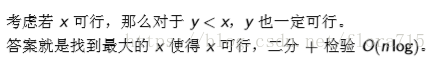### 4.纽约

https://www.luogu.org/problemnew/show/U33405

Azone 决定花费 w 元津巴布韦币，购买一辆载重为 w 的汽车。

Azone 每次出发前，会搬若干件总重不超过 w 的物品上车：出发前，车是空载的，

Azone 会选择能搬上车的家具中最重的一件放上车(即该家具之前还未运走且放置该家具后汽车不会超载），

Azone 希望在运送次数不超过 R的情况下完成转场，求 Azone 最少需要购置价值多少的车。#include<cstdio>
#include<iostream>
#include<algorithm>
#include<cmath>
#include<cstring>
using namespace std;

int n,R,a,l,r,mid;
int ans,pre,nxt;

bool okk(int w){
for(int i=1;i<=n;i++)
pre[i]=i-1,nxt[i]=i+1;
pre[n+1]=n; //链式用于记录仍留下的家具（已按价值排序过）
for(int i=1,s=0,x=n;s<n;x=pre[n+1],i++){
if(i>R) return false;
for(int p=w;p>0 && x;x=pre[x])
if(p>=a[x]){ //寻找最大可放家具
s++; p-=a[x];
nxt[pre[x]]=nxt[x];
pre[nxt[x]]=pre[x];
}
}
return true;
}

int main(){
scanf("%d%d",&n,&R);
for(int i=1;i<=n;i++){
scanf("%d",&a[i]);
r+=a[i],l=max(l,a[i]);
}
sort(a+1,a+1+n);
while(l<r){
mid=(l+r)>>1;
if(okk(mid)) r=mid;
else l=mid+1;
}
for(ans=l-50;ans<=l && !okk(ans);ans++);
printf("%d",ans);
return 0;
} 
View Code

——时间划过风的轨迹，那个少年，还在等你。

0
0 收藏

### 作者的其它热门文章0 评论
0 收藏
0